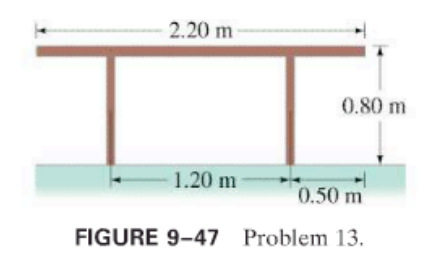×
Get Full Access to Physics: Principles With Applications - 6 Edition - Chapter 9 - Problem 13p
Get Full Access to Physics: Principles With Applications - 6 Edition - Chapter 9 - Problem 13p

×

# How close to the edge of the 20.0-kg table shown in Fig.ISBN: 9780130606204 3

## Solution for problem 13P Chapter 9

Physics: Principles with Applications | 6th Edition

• Textbook Solutions
• 2901 Step-by-step solutions solved by professors and subject experts
• Get 24/7 help from StudySoup virtual teaching assistantsPhysics: Principles with Applications | 6th Edition

4 5 1 395 Reviews
28
1
Problem 13P

How close to the edge of the 20.0-kg table shown in Fig. 9–47 can a 66.0-kg person sit without tipping it over?Step-by-Step Solution:

SOLUTION:Step 1 of 4The total momentum of system after collision is equal to the total momentum beforecollision.

Step 2 of 3

Step 3 of 3

## Discover and learn what students are asking

Calculus: Early Transcendental Functions : First-Order Linear Differential Equations
?In Exercises 5-14, solve the first-order linear differential equation. $$\frac{d y}{d x}+\left(\frac{2}{x}\right) y=3 x-5$$

Calculus: Early Transcendental Functions : Space Coordinates and Vectors in Space
?In Exercises 1-4, plot the points in the same three-dimensional coordinate system. (a) (2, 1, 3) (b) (-1, 2, 1)

Calculus: Early Transcendental Functions : Cylindrical and Spherical Coordinates
?In Exercises 1-6, convert the point from cylindrical coordinates to rectangular coordinates. $$(2,-\pi,-4)$$

Statistics: Informed Decisions Using Data : Data Collection
?What is meant by the process of statistics?

Unlock Textbook Solution